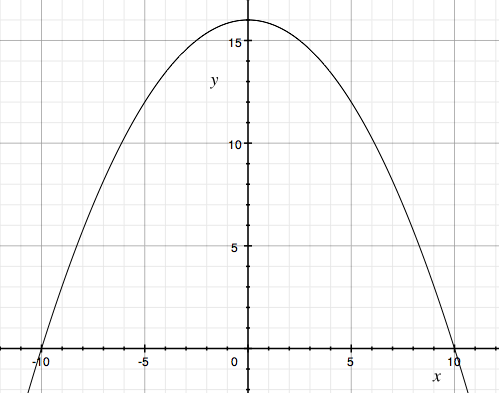SEARCH HOMEMath Central Quandaries & QueriesQuestion from Icyy: how high is the parabolic arch of span 20 feet and height of 16 feet, at a distance 5 feet from the center? what equation will I be going to use? thank youHi,

Since it is a parabolic arch it is parabola opening downwards. I am going to place the base on the X-axis and have it symmetric around the Y-axis. It will intersect the Y-axis at $y = 16$ and since the span is 20 feet it will intersect the X-axis at $x = -10, x = 10.$ Hence its graph looks likeParabolic Arch

Can you write its equation from this information?

PennyMath Central is supported by the University of Regina and The Pacific Institute for the Mathematical Sciences.